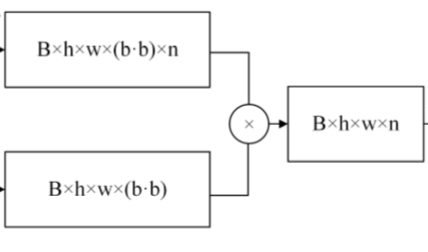# Pytorch: tensor multiplication

Hi!
I want to code this matrix multiplication:B=16, h=32, w=56, b*b=64, n=25
my code:

``````torch.mul(torch.rand([16, 32, 56, 64]),torch.rand([16, 32, 56, 64,25]))
``````

but I encountered in with this error:

``````The size of tensor a (64) must match the size of tensor b (25) at non-singleton dimension 4
``````

Would you mind helping me to fix this problem?

Hi,

I think the simplest is to write it as a matrix matrix multiplication. And so add a temporary dimension of size 1 on the first Tensor:

``````torch.matmul(torch.rand([16, 32, 56, 1, 64]),torch.rand([16, 32, 56, 64,25])).squeeze(-2)
``````
1 Like

It consider `Bxhxw` as batch dimensions. And `1x(bb)` and `(bb)xn` for the other dimensions of each other dimensions.
Doing the matrix multiply between the two, will give you a Tensor with `1xn` and with the same batch size.
Thanks for your help dear @albanD